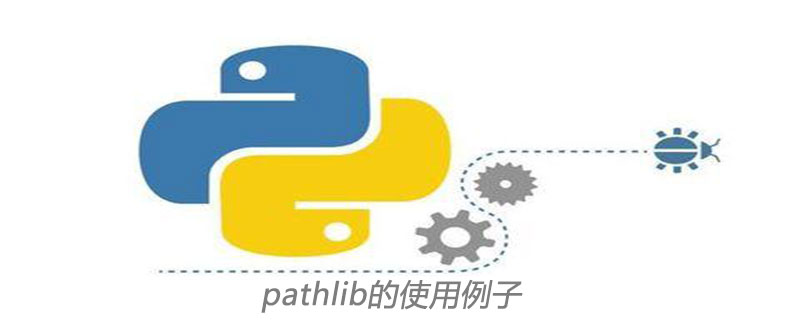Jquery中文网 www.jquerycn.cn
Jquery中文网 >  Python编程  >  Python入门  >  正文 pathlib的使用例子

# pathlib的使用例子

jquery中文网为您提供pathlib的使用例子等资源，欢迎您收藏本站，我们将为您提供最新的pathlib的使用例子资源```import pathlib
from collections import Counter
now_path = pathlib.Path.cwd()
gen = (i.suffix for i in now_path.iterdir())
print(Counter(gen))```

`Counter({'.py': 16, '': 11, '.txt': 1, '.png': 1, '.csv': 1})`

```import pathlib
from  collections import Counter
gen =(p.suffix for p in pathlib.Path.cwd().glob('*.py'))
print(Counter(gen))```

```import pathlib
from  collections import Counter
def tree(directory):
print(f'  {directory}')
for path in sorted(directory.rglob('*')):
depth = len(path.relative_to(directory).parts)
spacer = '    ' * depth
print(f'{spacer}  {path.name}')

now_path = pathlib.Path.cwd()

if __name__ == '__main__':
tree(now_path)```

parts方法可以返回路径的各部分。例如

```import pathlib
now_path = pathlib.Path.cwd()
if __name__ == '__main__':
print(now_path.parts)```

`('/', 'Users', 'chennan', 'pythonproject', 'demo')`

iterdir(),.glob()和.rglob()方法非常适合于生成器表达式和列表理解。

```import pathlib
now_path = pathlib.Path.cwd()
from datetime import datetime
time, file_path = max((f.stat().st_mtime, f) for f in now_path.iterdir())
print(datetime.fromtimestamp(time), file_path)```

```import pathlib
from datetime import datetime
now_path =pathlib.Path.cwd()
result = max((f.stat().st_mtime, f) for f in now_path.iterdir())

.stat().st_mtime会返回文件的时间戳，可以使用datetime或者time模块对时间格式进行进一步转换。

/运算符由__truediv__（）方法定义。 实际上，如果你看一下pathlib的源代码，你会看到类似的东西。

```class PurePath(object):

def __truediv__(self, key):
return self._make_child((key,))```
[]